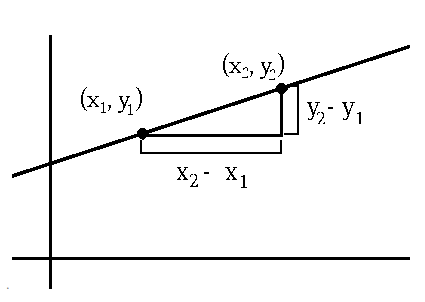# Slope Formula

The slope formula is used to calculate the steepness or the incline of a line. The x and y coordinates of the lines are used to calculate the slope of the lines. It is the ratio of the change in the y-axis to the change in the x-axis.## Formula of Slope

The formula to calculate slope is given as,

 $$\begin{array}{l}m=\frac{y_{2}-y_{1}}{x_{2}-x_{1}}\end{array}$$

Where m is the slope of the line. x1, x2 are the coordinates of x-axis and y1, y2 are the coordinates of y-axis

## Solved Examples

Question 1: Find the slope of a line whose coordinates are (2,7) and (8,1)?

Solution:

Given,

(x1, y1) = (2, 7)

(x2, y2) = (8, 1)

The slope formula is m = (y2 − y1 / x2 − x1)

m = (1 − 7/ 8 − 2)

m = −6/6

m = − 1

Question 2: If the slope of a line passing through the points (4, b) and (2, -9) is 3, then what is the value of b?

Solution:
Given,
Slope = m = 3
Points:
(x1 , y1 ) = (4, b)
(x2, y2) = (2, -9)
We know that,
Slope (m) = (y2– y1 )/(x2– x1)
3 = (-9 – b)/(2 – 4)
3 = (-9 – b)/(-2)
-9 – b = 3(-2)
-9 – b = -6
b = -9 + 6 = -3
Therefore, the value of b = -3.